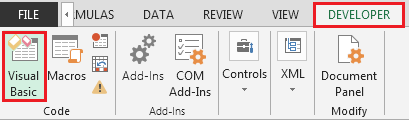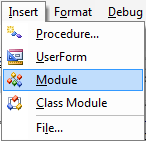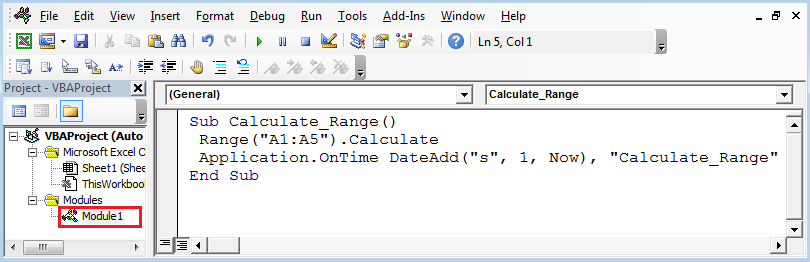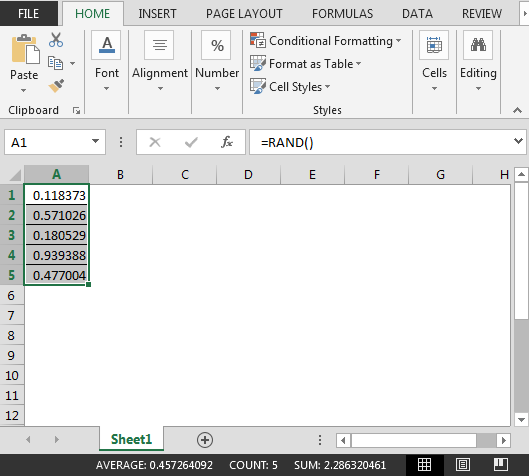Auto Refresh Excel Every 1 Second Using VBA in Excel

In this article, you will learn how to auto refresh excel file every 1 second using VBA code.

Question): In column A there are some values stored & I want them to automatically refresh every 1 second.

To refresh excel file every 1 second we will follow the below steps:

• In Range A1:A10; use RAND function
• We need to follow the below steps to launch VB editor
• Click on Developer tab
• From Code group select Visual Basic• Click on Insert then ModuleThis will create new module.

Enter the following code in the Module

Sub Calculate_Range()

Range("A1:A5").Calculate

End Sub• The above code has used the code application.ontime which is used to auto calculate the range A1:A5 to refresh every 1 second.
• Range A1:A5 contains RAND function which will keep on updating after every 1 secondIn this way we can schedule the excel file to refresh every x amount of time & save.

1.Many many thanks! saved me from lots of frustration....

2.can you let me know how to refresh time for every 1 second

•You can use OnTime events to refresh excel every 1 second.
sub RecalculateSheet()
application.calculate
end sub

sub calculculateEvery1Sec()
Application.ontime Now + (1 / 24 / 60 / 60), "RecalculateSheet"
end sub

Here is the brief and explanatory article about events in excel: Events in Excel VBA .

3.When I close the workbook that contains this VBA, it reopens automatically and according to the refresh interval stipulated. Only happens if I have another workbook open, as obviously that stops Excel from closing completely.

4.when i am trying to run it, it shows "syntax error"
5.•